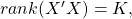We often see statements like “linear regression makes the assumption that the data is normally distributed”, “Data has no or little multicollinearity”, or other such blunders (you know who you are..).

Let’s set the whole thing straight.

It has to be said. Linear regression does not even assume linearity for that matter, I argue. It is simply an estimator, a function. We don’t need to ask anything from a function.

Consider that linear regression has an additional somewhat esoteric, geometric interpretation. When we perform a linear regression you simply find the best possible, closest possible, linear projection we can. A linear combination in your X space that is as close as possible in a Euclidean sense (squared distance) to some other vector y.

That is IT! a simple geometric relation. No assumptions needed whatsoever.

You don’t ask anything from the average when you use it as an estimate for the mean do you? So why do that when you use regression? We only need to ask more if we do something more.

## Why the confusion?

In my opinion, chief reason for the confusion is that we almost always perform a regression as a means to an end. We want to do something with those estimates. You know this- we want to show those mesmerizing asterisks. Often we attach the assumptions without giving it a second thought, if we need them or we do not, even when we are not busy with the dark art of upping t-values. Moreover, we are vindicated when we see that “everyone else is doing it”, and so the origin for those needlessly attached assumptions has somewhat faded over the years.

Paraphrasing ‘Don’t lose your temper until it’s time to lose your temper, you dig?’ (from the movie 25th Hour). Don’t Assume until you need to assume:

1. If you want to say that the linear combination you found is the conditional mean, then you need to assume a linear relation between X and y in the data.
2. If you want to say anything about the coefficients in small sample, whether they actually are different from zero then you need to assume normality.
You can also use bootstrap and relax that assumption.
3. If you want to say that you have used the ‘best linear unbiased estimator’ function for the conditional mean (BLUE, Gauss-Markov theorem) then you need a bunch of additional assumptions.

Now, if you don’t want to say anything about the distribution of the coefficients, or that you used the ‘best linear unbiased estimator’, you can altogether lose the term ‘normal’ and the assumptions that go with it.

Points 2 and 3 fork further confusion mind you. Solving a Gaussian Maximum-Likelihood (ML) estimation gives exactly the same solution as the one without any normality assumption. But it is not a matter of fact that ML estimation gives the same solution. It is a specific case of that particular Gaussian likelihood. Perhaps if the simple least square minimization would give rise to a different solution than that of the ML we would not be tangled like that.

## Illustration

To illustrate this point we can estimate the coefficients simply by finding the closest linear projection (minimizing the sum of squares), and compare it to that ML solution, under the normality assumption. They are the same, and both agree with the solution of the indispensable lm function.

First lets simulate some toy data: 100 observation with 3 explanatory variables with the relationand the variance of the residuals is 0.2^2.

## The code

Now we write the projection function and the likelihood function

Now we estimate the parameters

## Results

Actual Projection Gaussian Linear regression
coef_1 2 2.021 2.021 2.021
coef_2 0.5 0.497 0.497 0.497
coef_3 0.2 0.155 0.155 0.155
coef_4 0.1 0.086 0.086 0.086
SD_estimate 0.2 NA 0.208 0.209

For example if you are busy with forecasting using a regression, and you care about point forecasts only, you don’t need any additional assumptions. Call the assumptions when you need them. There is no need to unduly narrow your analysis.

1.Anjum says:

“linear regression makes the assumption that the data is normally distributed” – correct, OLS regression doesn’t make any such assumption.

But a regression will always pass through the mean of the data. That means that the regression is only an effective estimator if the (arithmetic) mean is the most likely value – i.e. if your data is normally distributed. If your data is log-normally distributed then the mean is often way off the most likely values. Also, taking the logarithm of your data, will ensure that the line passes through the geometric mean of the data – which may or may not be desirable.

2.tedthedog says:

The following post addressing a similar topic is recommended to dispel confusion:
http://varianceexplained.org/r/kmeans-free-lunch/

3.Plissken says:

The statement: “If you want to say anything about the coefficients, whether they actually are different from zero then you need to assume normality.” is wrong unless you are discussing small sample properties. You do not need to assume normality unless you want to test in small samples as asymptotic normality of the OLS estimator is provided by the CLT (Applies, in a time series setting, if we have: stationarity + weak dependence + contemporaneous exogeneity). Of course normality speeds up convergence and if we have normality then the OLS estimator is the same as the ML estimator but normality is not necessary in order to carry out inference on the estimated parameters.

Minimal conditions for identification of the OLS estimator are:
1)(contemporaneous exogeneity)
2)(where K is the number of explanatory variables and X is an NxK data matrix such thatis non-singular.)

Great blog by the way!

1.Eran Raviv says:

Fair enough, updated. Thanks.